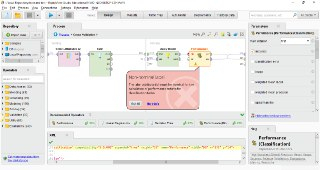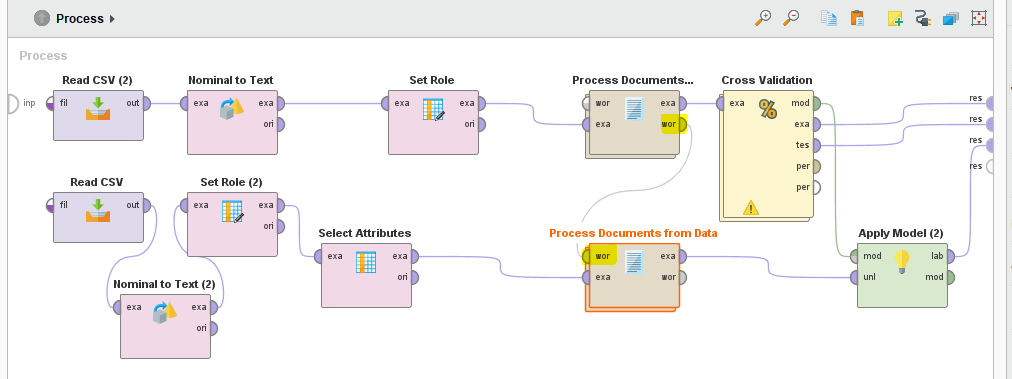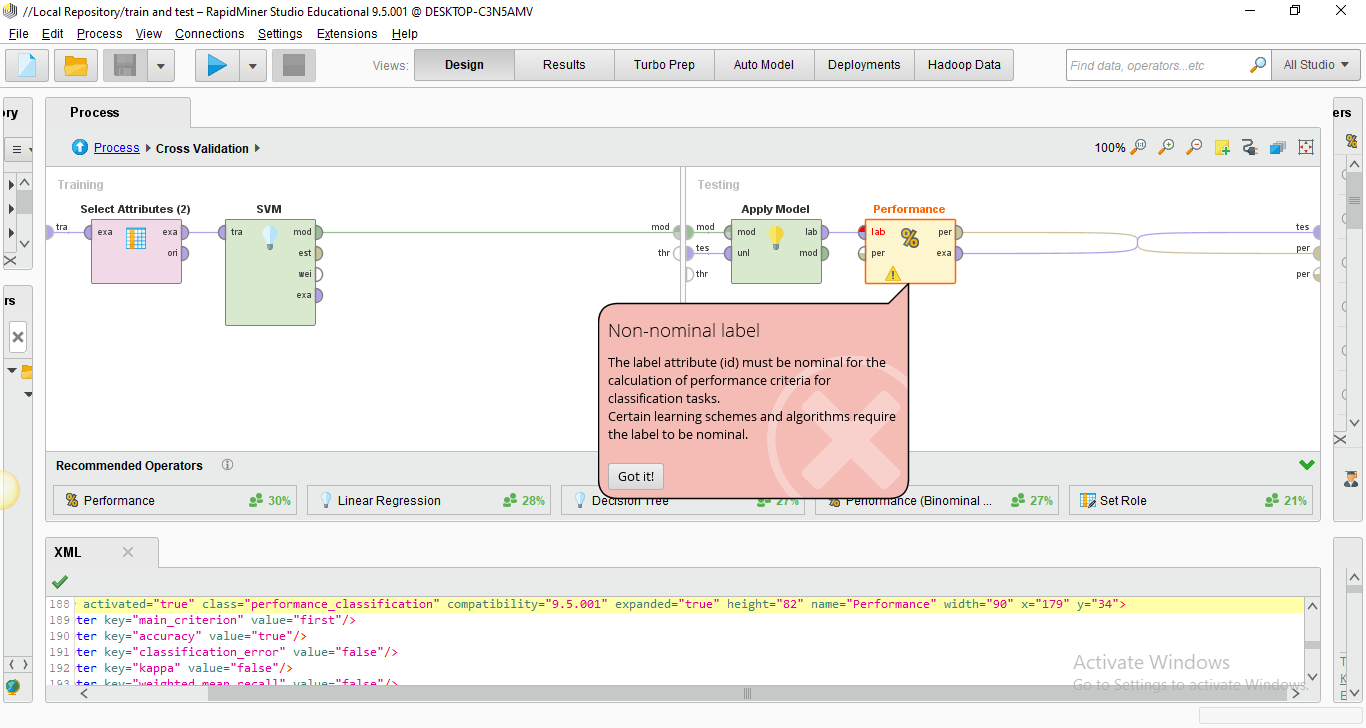# RapidMiner 9.7 is Now Available

Member Posts: 4Learner I
in Helpi have added the 'select attribute' operators to add the nominal attributes, id, label and all the attributes but still error keep going.• Administrator, Moderator, Employee, RMResearcher, Member Posts: 246RM Research
Hi @mims98 ,

can you share some more details about what you are trying to do with us? Your screenshot is too small to see any details. The best approach is copying and pasting the process XML in the forum.

Best,
David
• Member Posts: 4Learner I
Hello @David_A
here is the process XML. thanks for your response<?xml version="1.0" encoding="UTF-8"?><process version="9.5.001">
<context>
<input/>
<output/>
<macros/>
</context>
<operator activated="true" class="process" compatibility="9.5.001" expanded="true" name="Process">
<parameter key="logverbosity" value="init"/>
<parameter key="random_seed" value="2001"/>
<parameter key="send_mail" value="never"/>
<parameter key="process_duration_for_mail" value="30"/>
<parameter key="encoding" value="SYSTEM"/>
<process expanded="true">
<parameter key="csv_file" value="C:\Users\User\Desktop\train.csv"/>
<parameter key="column_separators" value=","/>
<parameter key="trim_lines" value="false"/>
<parameter key="use_quotes" value="true"/>
<parameter key="quotes_character" value="'"/>
<parameter key="escape_character" value="\"/>
<parameter key="comment_characters" value="#"/>
<parameter key="starting_row" value="1"/>
<parameter key="parse_numbers" value="true"/>
<parameter key="decimal_character" value="."/>
<parameter key="grouped_digits" value="false"/>
<parameter key="grouping_character" value=","/>
<parameter key="infinity_representation" value=""/>
<parameter key="date_format" value=""/>
<parameter key="first_row_as_names" value="true"/>
<list key="annotations"/>
<parameter key="time_zone" value="SYSTEM"/>
<parameter key="locale" value="English (United States)"/>
<parameter key="encoding" value="windows-1252"/>
<list key="data_set_meta_data_information">
<parameter key="0" value="id.true.integer.attribute"/>
<parameter key="1" value="label.true.integer.attribute"/>
<parameter key="2" value="tweet.true.polynominal.attribute"/>
</list>
<parameter key="datamanagement" value="double_array"/>
<parameter key="data_management" value="auto"/>
</operator>
<operator activated="true" class="read_csv" compatibility="9.5.001" expanded="true" height="68" name="Read CSV (2)" width="90" x="45" y="34">
<parameter key="csv_file" value="C:\Users\User\Desktop\test.csv"/>
<parameter key="column_separators" value=","/>
<parameter key="trim_lines" value="false"/>
<parameter key="use_quotes" value="true"/>
<parameter key="quotes_character" value="&quot;"/>
<parameter key="escape_character" value="\"/>
<parameter key="comment_characters" value="#"/>
<parameter key="starting_row" value="1"/>
<parameter key="parse_numbers" value="true"/>
<parameter key="decimal_character" value="."/>
<parameter key="grouped_digits" value="false"/>
<parameter key="grouping_character" value=","/>
<parameter key="infinity_representation" value=""/>
<parameter key="date_format" value=""/>
<parameter key="first_row_as_names" value="true"/>
<list key="annotations"/>
<parameter key="time_zone" value="SYSTEM"/>
<parameter key="locale" value="English (United States)"/>
<parameter key="encoding" value="windows-1252"/>
<list key="data_set_meta_data_information">
<parameter key="0" value="id.true.integer.attribute"/>
<parameter key="1" value="tweet.true.polynominal.attribute"/>
</list>
<parameter key="datamanagement" value="double_array"/>
<parameter key="data_management" value="auto"/>
</operator>
<operator activated="true" class="nominal_to_text" compatibility="9.5.001" expanded="true" height="82" name="Nominal to Text" width="90" x="179" y="34">
<parameter key="attribute_filter_type" value="all"/>
<parameter key="attribute" value=""/>
<parameter key="attributes" value=""/>
<parameter key="use_except_expression" value="false"/>
<parameter key="value_type" value="nominal"/>
<parameter key="use_value_type_exception" value="false"/>
<parameter key="except_value_type" value="file_path"/>
<parameter key="block_type" value="single_value"/>
<parameter key="use_block_type_exception" value="false"/>
<parameter key="except_block_type" value="single_value"/>
<parameter key="invert_selection" value="false"/>
<parameter key="include_special_attributes" value="false"/>
</operator>
<operator activated="true" class="set_role" compatibility="9.5.001" expanded="true" height="82" name="Set Role" width="90" x="380" y="34">
<parameter key="attribute_name" value="id"/>
<parameter key="target_role" value="label"/>
</operator>
<operator activated="true" class="text:process_document_from_data" compatibility="8.2.000" expanded="true" height="82" name="Process Documents from Data (2)" width="90" x="581" y="34">
<parameter key="create_word_vector" value="true"/>
<parameter key="vector_creation" value="TF-IDF"/>
<parameter key="keep_text" value="false"/>
<parameter key="prune_method" value="none"/>
<parameter key="prune_below_percent" value="3.0"/>
<parameter key="prune_above_percent" value="30.0"/>
<parameter key="prune_below_rank" value="0.05"/>
<parameter key="prune_above_rank" value="0.95"/>
<parameter key="datamanagement" value="double_sparse_array"/>
<parameter key="data_management" value="auto"/>
<parameter key="select_attributes_and_weights" value="false"/>
<list key="specify_weights"/>
<process expanded="true">
<operator activated="false" class="text:tokenize" compatibility="8.2.000" expanded="true" height="68" name="Tokenize" width="90" x="45" y="34">
<parameter key="mode" value="non letters"/>
<parameter key="characters" value=".:"/>
<parameter key="language" value="English"/>
<parameter key="max_token_length" value="3"/>
</operator>
<operator activated="false" class="text:transform_cases" compatibility="8.2.000" expanded="true" height="68" name="Transform Cases (2)" width="90" x="179" y="34">
<parameter key="transform_to" value="lower case"/>
</operator>
<operator activated="false" class="text:filter_stopwords_english" compatibility="8.2.000" expanded="true" height="68" name="Filter Stopwords (English) (2)" width="90" x="380" y="34"/>
<connect from_port="document" to_port="document 1"/>
<portSpacing port="source_document" spacing="0"/>
<portSpacing port="sink_document 1" spacing="0"/>
<portSpacing port="sink_document 2" spacing="0"/>
</process>
</operator>
<operator activated="true" class="concurrency:cross_validation" compatibility="9.5.001" expanded="true" height="145" name="Cross Validation" width="90" x="715" y="34">
<parameter key="split_on_batch_attribute" value="false"/>
<parameter key="leave_one_out" value="false"/>
<parameter key="number_of_folds" value="10"/>
<parameter key="sampling_type" value="automatic"/>
<parameter key="use_local_random_seed" value="false"/>
<parameter key="local_random_seed" value="1992"/>
<parameter key="enable_parallel_execution" value="true"/>
<process expanded="true">
<operator activated="true" class="select_attributes" compatibility="9.5.001" expanded="true" height="82" name="Select Attributes (2)" width="90" x="45" y="34">
<parameter key="attribute_filter_type" value="all"/>
<parameter key="attribute" value=""/>
<parameter key="attributes" value=""/>
<parameter key="use_except_expression" value="false"/>
<parameter key="value_type" value="integer"/>
<parameter key="use_value_type_exception" value="true"/>
<parameter key="except_value_type" value="text"/>
<parameter key="block_type" value="attribute_block"/>
<parameter key="use_block_type_exception" value="false"/>
<parameter key="except_block_type" value="value_matrix_row_start"/>
<parameter key="invert_selection" value="false"/>
<parameter key="include_special_attributes" value="true"/>
</operator>
<operator activated="true" class="support_vector_machine" compatibility="9.5.001" expanded="true" height="124" name="SVM" width="90" x="179" y="34">
<parameter key="kernel_type" value="polynomial"/>
<parameter key="kernel_gamma" value="1.0"/>
<parameter key="kernel_sigma1" value="1.0"/>
<parameter key="kernel_sigma2" value="0.0"/>
<parameter key="kernel_sigma3" value="2.0"/>
<parameter key="kernel_shift" value="1.0"/>
<parameter key="kernel_degree" value="2.0"/>
<parameter key="kernel_a" value="1.0"/>
<parameter key="kernel_b" value="0.0"/>
<parameter key="kernel_cache" value="200"/>
<parameter key="C" value="0.0"/>
<parameter key="convergence_epsilon" value="0.001"/>
<parameter key="max_iterations" value="100000"/>
<parameter key="scale" value="true"/>
<parameter key="calculate_weights" value="true"/>
<parameter key="return_optimization_performance" value="true"/>
<parameter key="L_pos" value="1.0"/>
<parameter key="L_neg" value="1.0"/>
<parameter key="epsilon" value="0.0"/>
<parameter key="epsilon_plus" value="0.0"/>
<parameter key="epsilon_minus" value="0.0"/>
<parameter key="balance_cost" value="false"/>
<parameter key="estimate_performance" value="false"/>
</operator>
<connect from_port="training set" to_op="Select Attributes (2)" to_port="example set input"/>
<connect from_op="Select Attributes (2)" from_port="example set output" to_op="SVM" to_port="training set"/>
<connect from_op="SVM" from_port="model" to_port="model"/>
<portSpacing port="source_training set" spacing="0"/>
<portSpacing port="sink_model" spacing="0"/>
<portSpacing port="sink_through 1" spacing="0"/>
</process>
<process expanded="true">
<operator activated="true" class="apply_model" compatibility="9.5.001" expanded="true" height="82" name="Apply Model" width="90" x="45" y="34">
<list key="application_parameters"/>
<parameter key="create_view" value="false"/>
</operator>
<operator activated="true" class="performance_classification" compatibility="9.5.001" expanded="true" height="82" name="Performance" width="90" x="179" y="34">
<parameter key="main_criterion" value="first"/>
<parameter key="accuracy" value="true"/>
<parameter key="classification_error" value="false"/>
<parameter key="kappa" value="false"/>
<parameter key="weighted_mean_recall" value="false"/>
<parameter key="weighted_mean_precision" value="false"/>
<parameter key="spearman_rho" value="false"/>
<parameter key="kendall_tau" value="false"/>
<parameter key="absolute_error" value="false"/>
<parameter key="relative_error" value="false"/>
<parameter key="relative_error_lenient" value="false"/>
<parameter key="relative_error_strict" value="false"/>
<parameter key="normalized_absolute_error" value="false"/>
<parameter key="root_mean_squared_error" value="false"/>
<parameter key="root_relative_squared_error" value="false"/>
<parameter key="squared_error" value="false"/>
<parameter key="correlation" value="false"/>
<parameter key="squared_correlation" value="false"/>
<parameter key="cross-entropy" value="false"/>
<parameter key="margin" value="false"/>
<parameter key="soft_margin_loss" value="false"/>
<parameter key="logistic_loss" value="false"/>
<parameter key="skip_undefined_labels" value="true"/>
<parameter key="use_example_weights" value="true"/>
<list key="class_weights"/>
</operator>
<connect from_port="model" to_op="Apply Model" to_port="model"/>
<connect from_port="test set" to_op="Apply Model" to_port="unlabelled data"/>
<connect from_op="Apply Model" from_port="labelled data" to_op="Performance" to_port="labelled data"/>
<connect from_op="Performance" from_port="performance" to_port="performance 1"/>
<connect from_op="Performance" from_port="example set" to_port="test set results"/>
<portSpacing port="source_model" spacing="0"/>
<portSpacing port="source_test set" spacing="0"/>
<portSpacing port="source_through 1" spacing="0"/>
<portSpacing port="sink_test set results" spacing="0"/>
<portSpacing port="sink_performance 1" spacing="0"/>
<portSpacing port="sink_performance 2" spacing="0"/>
</process>
</operator>
<operator activated="true" class="nominal_to_text" compatibility="9.5.001" expanded="true" height="82" name="Nominal to Text (2)" width="90" x="112" y="238">
<parameter key="attribute_filter_type" value="all"/>
<parameter key="attribute" value=""/>
<parameter key="attributes" value=""/>
<parameter key="use_except_expression" value="false"/>
<parameter key="value_type" value="nominal"/>
<parameter key="use_value_type_exception" value="false"/>
<parameter key="except_value_type" value="file_path"/>
<parameter key="block_type" value="single_value"/>
<parameter key="use_block_type_exception" value="false"/>
<parameter key="except_block_type" value="single_value"/>
<parameter key="invert_selection" value="false"/>
<parameter key="include_special_attributes" value="false"/>
</operator>
<operator activated="true" class="set_role" compatibility="9.5.001" expanded="true" height="82" name="Set Role (2)" width="90" x="179" y="136">
<parameter key="attribute_name" value="tweet"/>
<parameter key="target_role" value="label"/>
<parameter key="label" value="regular"/>
</list>
</operator>
<operator activated="true" class="select_attributes" compatibility="9.5.001" expanded="true" height="82" name="Select Attributes" width="90" x="313" y="187">
<parameter key="attribute_filter_type" value="all"/>
<parameter key="attribute" value="label"/>
<parameter key="attributes" value=""/>
<parameter key="use_except_expression" value="false"/>
<parameter key="value_type" value="attribute_value"/>
<parameter key="use_value_type_exception" value="false"/>
<parameter key="except_value_type" value="time"/>
<parameter key="block_type" value="attribute_block"/>
<parameter key="use_block_type_exception" value="false"/>
<parameter key="except_block_type" value="value_matrix_row_start"/>
<parameter key="invert_selection" value="false"/>
<parameter key="include_special_attributes" value="true"/>
</operator>
<operator activated="true" class="text:process_document_from_data" compatibility="8.2.000" expanded="true" height="82" name="Process Documents from Data" width="90" x="447" y="187">
<parameter key="create_word_vector" value="true"/>
<parameter key="vector_creation" value="TF-IDF"/>
<parameter key="keep_text" value="false"/>
<parameter key="prune_method" value="none"/>
<parameter key="prune_below_percent" value="3.0"/>
<parameter key="prune_above_percent" value="30.0"/>
<parameter key="prune_below_rank" value="0.05"/>
<parameter key="prune_above_rank" value="0.95"/>
<parameter key="datamanagement" value="double_sparse_array"/>
<parameter key="data_management" value="auto"/>
<parameter key="select_attributes_and_weights" value="false"/>
<list key="specify_weights"/>
<process expanded="true">
<operator activated="true" class="text:tokenize" compatibility="8.2.000" expanded="true" height="68" name="Tokenize (2)" width="90" x="45" y="34">
<parameter key="mode" value="non letters"/>
<parameter key="characters" value=".:"/>
<parameter key="language" value="English"/>
<parameter key="max_token_length" value="3"/>
</operator>
<operator activated="true" class="text:transform_cases" compatibility="8.2.000" expanded="true" height="68" name="Transform Cases" width="90" x="179" y="34">
<parameter key="transform_to" value="lower case"/>
</operator>
<operator activated="false" class="text:filter_stopwords_english" compatibility="8.2.000" expanded="true" height="68" name="Filter Stopwords (English)" width="90" x="313" y="85"/>
<connect from_port="document" to_op="Tokenize (2)" to_port="document"/>
<connect from_op="Tokenize (2)" from_port="document" to_op="Transform Cases" to_port="document"/>
<connect from_op="Transform Cases" from_port="document" to_port="document 1"/>
<portSpacing port="source_document" spacing="0"/>
<portSpacing port="sink_document 1" spacing="0"/>
<portSpacing port="sink_document 2" spacing="0"/>
</process>
</operator>
<operator activated="true" class="apply_model" compatibility="9.5.001" expanded="true" height="82" name="Apply Model (2)" width="90" x="715" y="187">
<list key="application_parameters"/>
<parameter key="create_view" value="false"/>
</operator>
<connect from_op="Read CSV" from_port="output" to_op="Nominal to Text (2)" to_port="example set input"/>
<connect from_op="Read CSV (2)" from_port="output" to_op="Nominal to Text" to_port="example set input"/>
<connect from_op="Nominal to Text" from_port="example set output" to_op="Set Role" to_port="example set input"/>
<connect from_op="Set Role" from_port="example set output" to_op="Process Documents from Data (2)" to_port="example set"/>
<connect from_op="Process Documents from Data (2)" from_port="example set" to_op="Cross Validation" to_port="example set"/>
<connect from_op="Cross Validation" from_port="model" to_op="Apply Model (2)" to_port="model"/>
<connect from_op="Cross Validation" from_port="example set" to_port="result 1"/>
<connect from_op="Cross Validation" from_port="test result set" to_port="result 2"/>
<connect from_op="Nominal to Text (2)" from_port="example set output" to_op="Set Role (2)" to_port="example set input"/>
<connect from_op="Set Role (2)" from_port="example set output" to_op="Select Attributes" to_port="example set input"/>
<connect from_op="Select Attributes" from_port="example set output" to_op="Process Documents from Data" to_port="example set"/>
<connect from_op="Process Documents from Data" from_port="example set" to_op="Apply Model (2)" to_port="unlabelled data"/>
<connect from_op="Apply Model (2)" from_port="labelled data" to_port="result 3"/>
<portSpacing port="source_input 1" spacing="0"/>
<portSpacing port="sink_result 1" spacing="0"/>
<portSpacing port="sink_result 2" spacing="0"/>
<portSpacing port="sink_result 3" spacing="0"/>
<portSpacing port="sink_result 4" spacing="0"/>
</process>
</operator>
</process>

• Administrator, Employee, RapidMiner Certified Analyst, RapidMiner Certified Expert, Member Posts: 273RM Data Scientist
Hi @mims98,
Thanks for sharing the process. The "Nominal to text" was applied to all attributes. Do you want to apply text processing on all attributes? Usually, I only need to apply the nominal to text conversion on one single column(nominal attribute).
One more potential issue is that there is new keywords in the second input data. So I would suggest using the "wordlist" from the first input data, and reuse this wordlist on second data.Hope it helps.

YY
• Administrator, Moderator, Employee, RMResearcher, Member Posts: 246RM Research
Hi,

I can't run the process, because I don't have your CSV. Could you please provide with a clearer picture or description what the error says?

What I also saw in your process, that in the first Process Documents from Data operator, all operators all operators are disabled. To apply the model on a test set, you need to apply the same transformations on both data sets.

Best,
David
• Member Posts: 4Learner I
edited June 27
@yyhuang
thank you, I have try to change it on one single column only. but it going to be error for my  "SVM" operator which is  "missing attribute"
where I should put the  "wordlist" operator?

• Member Posts: 4Learner I
@David_Aerror at performance operator :
non-nominal label
the label attribute(id) must be nominal for the calculation of performance criteria for classification tasks. certain learning schemes and algorithms require the label to be nominal

here is the data in CSV:

• Administrator, Moderator, Employee, RMResearcher, Member Posts: 246RM Research
Okay,

the technical reason your process fails is, because you are trying to predict the ID represented as a number and the performance measure you apply are used for categories. Using the Numerical to Polynominal would help you in that case.
But in your data the IDs are unique and so can't be used as labels for a classification problem (how should an algorithm learn to detect the right label, if each only appears one time). So perhaps you need to rephrase your learning task first and then update your process accordingly.
Perhaps take a look at the community or the RapidMiner Academy for some inspiration on different text classification tasks.

Best,
David